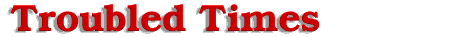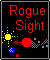#Equation

The equation proceeds as follows:

LN [9.362 / 6.8424] / 9.7 = k
LN [1.368233369]/9.7 = k
0.313520396 /9.7 = k
0.03232169 = k the rate of change in velocity

Now that the variable (k), the rate of acceleration of the 12th planet is known, we can use the same equation to solve for the distance between the Sun and the 12th planet at any variable time (t). Since we are solving for a distance from the Sun to the 12th planet prior to Sun passage, time (t) takes on a negative factor. So the final equation looks like: Distance(final) = Distance(initial) * e ^ [(k) * (-t)]. Substituting known values, Distance(final), the distance from the Sun to the 12th planet is the unknown. Distance(initial) is equal to 9.362 units, because we are solving for a distance prior to the passage in the year 2003. This would be equal to the total distance from the midpoint to the Sun. The decimal 0.03232169 is equal to (k) the acceleration of the 12th planet, acquired from the previous calculation.

The variable time (t) can have a range from 0 to about 47,000 weeks prior to Sun passage. Distance(final) at some designated time (t), will be a percentage of Distance(initial) the full distance between Sun and the mid-point that the 12th planet has traveled towards the Sun. To find Distance(final), multiply the (k) value by a negative (t) value measured in weeks. Look up or calculate the equivalent e value for the decimal, and then multiply it by 9.362. This will give you the distance traveled by the 12th planet from the mid point towards the Sun at a designated time (t). To get the distance from the 12th planet to Earth orbit, subtract [Earth/Sun distance .025293 S-P units (92.95 million miles) and the total distance traveled by the 12th planet from the mid point at designated time(t) acquired from the above equation], from 9.362 units, the total distance between the mid point and the Sun. For informational purposes 1 S-P unit is equal to 3.675 billion miles. The distance table will give an approximate distance to the 12th planet and a point where it pierces Earth's orbital path on the near side of the sun.

Offered by Robert.

I'm not a mathematician or anything, but my one friend who works in astrophysics has told me that the equation for this page is wrong. As I said, I'm no math expert, but you might want to look over this and make any appropriate corrections. Here is what he wrote.

The equation says that the distance of the planet can be described by an exponential function,
distance(final)=distance(initial)*e^(k*t),
where k is the change in velocity. however, anything in an exponent has to be dimensionless, i.e. no units. Therefore if t is time, the units of k must be inverse time, or frequency. Not acceleration, which is distance per time squared. And the rate of change of velocity, or acceleration, would just be the 2nd derivative of the distance equation. Or,
accel = distance(initial)*k^2*e^(k*t).

Offered by Andrew.